# 1754 and a Level 2 Puzzle

### Today’s Puzzle:

Can you write each number from 1 to 12 in the first column and again in the top row so that the products of the numbers you write are the given clues?### Factors of 1754:

• 1754 is a composite number.
• Prime factorization: 1754 = 2 × 877.
• 1754 has no exponents greater than 1 in its prime factorization, so √1754 cannot be simplified.
• The exponents in the prime factorization are 1 and 1. Adding one to each exponent and multiplying we get (1 + 1)(1 + 1) = 2 × 2 = 4. Therefore 1754 has exactly 4 factors.
• The factors of 1754 are outlined with their factor pair partners in the graphic below.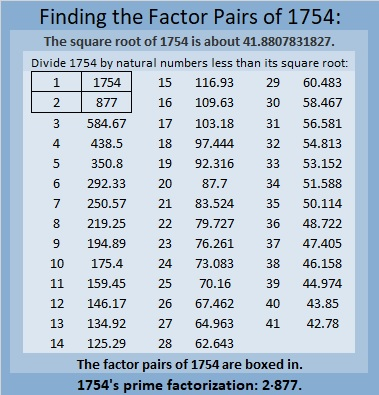### More About the Number 1754:

1754 is the sum of two squares:
35² + 23² = 1754.

1754 is the hypotenuse of a Pythagorean triple:
696-1610-1754, calculated from 35² – 23², 2(35)(23), 35² + 23².
It is also 2 times (348-805-877).

# 1752 So Many Snowflakes

### Today’s Puzzle:

A winter storm hit much of the United States today including the drought-stricken area where I live. We are so grateful for the snow, but also grateful we could stay home all day. Driving in this much snow is not much fun.

This snowflake mystery-level puzzle might have a couple of tricky clues, but I’m confident that you’ll still find it easier than clearing a couple of feet of snow off a driveway! Just write the numbers 1 to 12 in both the first column and the top row so that those numbers and the given clues make a multiplication table.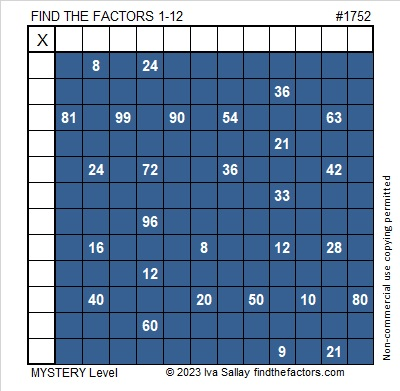Here’s the same puzzle without any added color: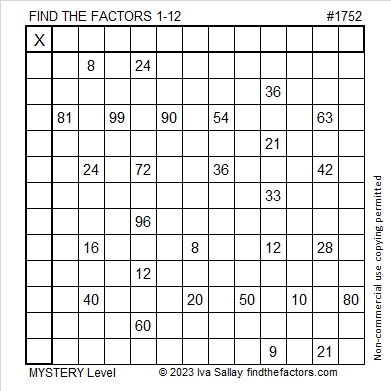### Factors of 1752:

• 1752 is a composite number.
• Prime factorization: 1752 = 2 × 2 × 2 × 3 × 73, which can be written 1752 = 2³ × 3 × 73.
• 1752 has at least one exponent greater than 1 in its prime factorization so √1752 can be simplified. Taking the factor pair from the factor pair table below with the largest square number factor, we get √1752 = (√4)(√438) = 2√438.
• The exponents in the prime factorization are 3,1 and 1. Adding one to each exponent and multiplying we get (3 + 1)(1 + 1) (1 + 1) = 4 × 2 × 2 = 16. Therefore 1752 has exactly 16 factors.
• The factors of 1752 are outlined with their factor pair partners in the graphic below.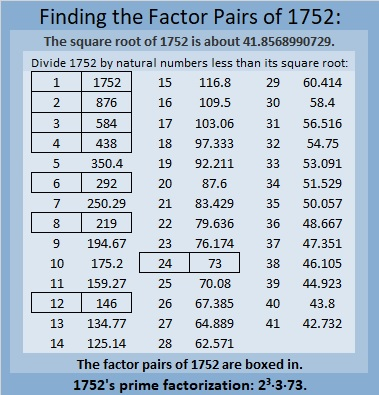### More About the Number 1752:

1752 is the hypotenuse of a Pythagorean triple:
1152 1320 1752, which is 24 times (48-55-73).

1752 is the difference of two squares in FOUR different ways:
439² – 437² = 1752,
221² – 217² = 1752,
149² -143² = 1752, and
79² – 67² = 1752.

1752 is a palindrome in some other bases:
It’s A4A in base13 because 10(13²) + 4(13) + 10(1) = 1752,
it’s 4G4 in base19 because 4(19²) + 16(19) + 4(1) = 1752, and
it’s 2K2 in base 25 because 2(25²) + 20(25) + 2(1) = 1752.

# 1751 A Pythagorean Triple Puzzle

### Today’s Puzzle:

Even though they don’t look like it, the fourteen triangles in today’s puzzle are all Pythagorean triple triangles that most certainly have not been drawn to scale. The marked angle is the right angle for each of triangle. The hypotenuse of each triangle is less than 100. There is a chart under the puzzle listing all possible Pythagorean triples that could be used in the puzzle. No triple will be used more than once. Use logic to figure out what the missing side lengths are. Have fun!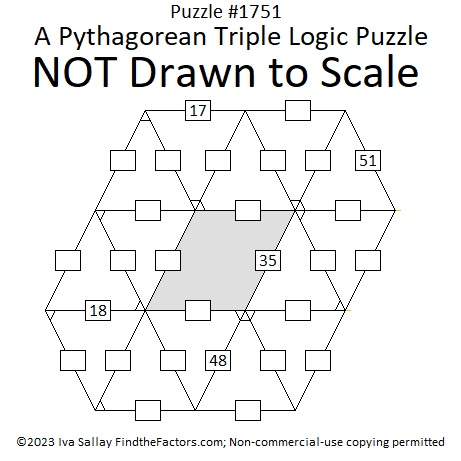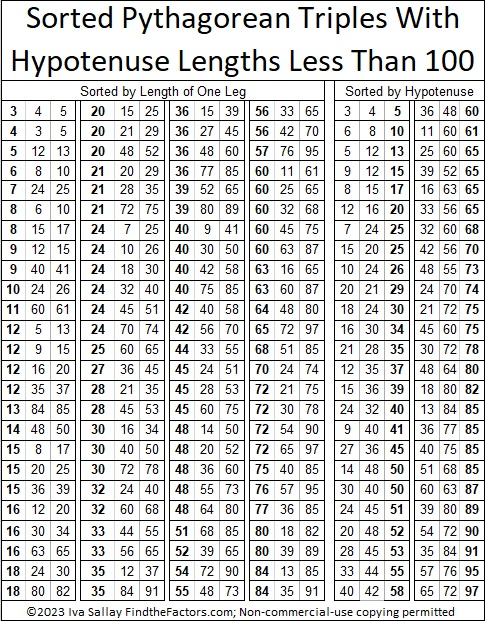### Factors of 1751:

If you remember that 51 is NOT a prime number because 3 × 17 = 51, then it isn’t difficult to recognize that 1751 also must be divisible by 17.

• 1751 is a composite number.
• Prime factorization: 1751 = 17 × 103.
• 1751 has no exponents greater than 1 in its prime factorization, so √1751 cannot be simplified.
• The exponents in the prime factorization are 1 and 1. Adding one to each exponent and multiplying we get (1 + 1)(1 + 1) = 2 × 2 = 4. Therefore 1751 has exactly 4 factors.
• The factors of 1751 are outlined with their factor pair partners in the graphic below.### More About the Number 1751:

1751 is the hypotenuse of a Pythagorean triple:
824-1545-1751 which is (8-15-17) times 103.

1751 looks interesting in some other bases:
It’s 575 in base 18 because 5(18²) + 7(18) + 5(1) = 1751,
373 base 23 because 3(23²) + 7(23) + 3(1) = 1751, and
1F1 base 35 because 1(35²) + 15(35) + 1(1) = 1751.

# 1750 and Level 1

### Today’s Puzzle:

Place the numbers 1 to 12 in the first column and also in the top row so that those numbers are the factors of the given clues.### Factors of 1750:

• 1750 is a composite number.
• Prime factorization: 1750 = 2 × 5 × 5 × 5 × 7, which can be written 1750 = 2 × 5³ × 7.
• 1750 has at least one exponent greater than 1 in its prime factorization so √1750 can be simplified. Taking the factor pair from the factor pair table below with the largest square number factor, we get √1750 = (√25)(√70) = 5√70.
• The exponents in the prime factorization are 1,3 and 1. Adding one to each exponent and multiplying we get (1 + 1)(3 + 1)(1 + 1) = 2 × 4 × 2 = 16. Therefore 1750 has exactly 16 factors.
• The factors of 1750 are outlined with their factor pair partners in the graphic below.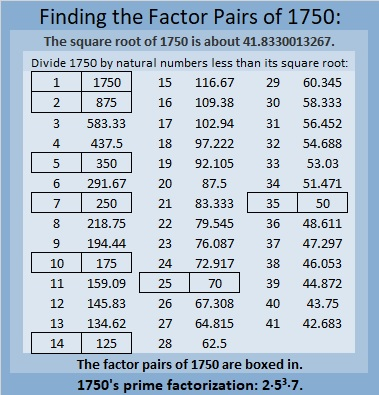### More About the Number 1750:

1750 is the hypotenuse of THREE Pythagorean triples:
490 1680 1750, which is (7-24-25) times 70,
616 1638 1750, which is 14 times (44-117-125), and
1050 1400 1750, which is (3-4-5) times 350.

1750 looks interesting in some other bases:
It’s 5050 in base 7 because 5(7³) + 5(7) = 1750, and it’s
1K1 in base 33 because 1(33²) + 20(33) + 1(1) = 1750.

# 1749 Happy Valentine’s Day!

### Today’s Puzzle:

Here’s one more Valentine’s puzzle for you to do. Enjoy!### Factors of 1749:

1749 is divisible by 3 because 7 is half of 14 so any 3-digit number made from the digits 714 in any order is divisible by 3, and of course, 9 is divisible by 3.

1749 is divisible by 11 because 1 – 7 + 4 – 9 = -11, a multiple of 11.

• 1749 is a composite number.
• Prime factorization: 1749 = 3 × 11 × 53.
• 1749 has no exponents greater than 1 in its prime factorization, so √1749 cannot be simplified.
• The exponents in the prime factorization are 1, 1, and 1. Adding one to each exponent and multiplying we get (1 + 1)(1 + 1)(1 + 1) = 2 × 2 × 2 = 8. Therefore 1749 has exactly 8 factors.
• The factors of 1749 are outlined with their factor pair partners in the graphic below.### More About the Number 1749:

1749 is the hypotenuse of a Pythagorean triple:
924-1485-1749 which is 33 times (28-45-53).

1749 is the difference of two squares in FOUR different ways:
875² – 874² = 1749,
293² – 290² = 1749,
85² – 74² = 1749, and
43² – 10² = 1749.

# 1748 A Factor Fits Valentine’s Puzzle

### Today’s Puzzle:

Can you find the one and only solution to this Factor Fits Valentine puzzle or will it give you fits?### Factors of 1748:

• 1748 is a composite number.
• Prime factorization: 1748 = 2 × 2 × 19 × 23, which can be written 1748 = 2² × 19 × 23.
• 1748 has at least one exponent greater than 1 in its prime factorization so √1748 can be simplified. Taking the factor pair from the factor pair table below with the largest square number factor, we get √1748 = (√4)(√437) = 2√437.
• The exponents in the prime factorization are 2, 1, and 1. Adding one to each exponent and multiplying we get (2 + 1)(1 + 1)(1 + 1) = 3 × 2 × 2 = 12. Therefore 1748 has exactly 12 factors.
• The factors of 1748 are outlined with their factor pair partners in the graphic below.### More About the Number 1748:

1748 is the difference of two squares in two ways:
438² – 436² = 1748, and
42² – 4² = 1748.
That means we are only 16 numbers away from the next perfect square!

# 1747 Getting a Super Bowl Ring Isn’t Easy

### Today’s Puzzle:

On Wednesday I read an article in my local paper titled From undrafted free agent to the biggest stage: Britain Covey’s ‘unique ride’ to Super Bowl LVII. It got me quite excited for today’s game. Unfortunately, between Wednesday and Sunday, Covey suffered a hamstring injury.  Getting a Super Bowl Ring certainly isn’t easy. Being able to contribute to your team’s winning the game isn’t easy either. UPDATE: Good News! He was able to make at least one play in the second quarter! Further update: Even though his team lost the game in the final minutes, this rookie played well when he was on the field. I think both teams played exceptionally well and gave us all an exciting game to watch.

Before the game, it is a mystery which team will win the game. The difficulty level of this puzzle is a mystery also.

If you look at today’s puzzle just right, I think it looks a little like a super bowl ring, but I forwarn you, it will not be easy to get this puzzle either. You will need to place the numbers from 1 to 12 both in the first column and in the top row so that the given clues are the products of the numbers you place. Logic and practice will get you there. Good Luck!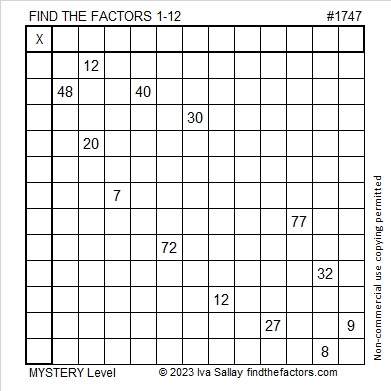You also might enjoy this next Super Bowl puzzle I saw on Twitter:

### Factors of 1747:

• 1747 is a prime number.
• Prime factorization: 1747 is prime.
• 1747 has no exponents greater than 1 in its prime factorization, so √1747 cannot be simplified.
• The exponent in the prime factorization is 1. Adding one to that exponent we get (1 + 1) = 2. Therefore 1747 has exactly 2 factors.
• The factors of 1747 are outlined with their factor pair partners in the graphic below.How do we know that 1747 is a prime number? If 1747 were not a prime number, then it would be divisible by at least one prime number less than or equal to √1747. Since 1747 cannot be divided evenly by 2, 3, 5, 7, 11, 13, 17, 19, 23, 29, 31, 37, or 41, we know that 1747 is a prime number.

### More About the Number 1747:

1747 is a palindrome in base 15.
It’s 7B7₁₅ because 7(15²) + 11(15) + 7(1) = 1747.

# 1746 Love-Struck with Multiplication

### Today’s Puzzle:

If you solve this Valentine’s multiplication table puzzle, you might just become love-struck with multiplication! It’s a level 6 puzzle so you might find most of the clues to be tricky. Be sure to use logic as you place each number from 1 to 10 in both the first column and also in the top row. There is only one solution.### Factors of 1746:

• 1746 is a composite number.
• Prime factorization: 1746 = 2 × 3 × 3 × 97, which can be written 1746 = 2 × 3² × 97.
• 1746 has at least one exponent greater than 1 in its prime factorization so √1746 can be simplified. Taking the factor pair from the factor pair table below with the largest square number factor, we get √1746 = (√9)(√194) = 3√194.
• The exponents in the prime factorization are 1, 2, and 1. Adding one to each exponent and multiplying we get (1 + 1)(2 + 1)(1 + 1) = 2 × 3 × 2 = 12. Therefore 1746 has exactly 12 factors.
• The factors of 1746 are outlined with their factor pair partners in the graphic below.### More About the Number 1746:

Why is 1746 the sum of two squares? We can tell by looking at its PRIME factors:
2 is a power of 2,
3² is an even power, and
97 is a prime Pythagorean hypotenuse. (It is prime and one more than a positive multiple of 4).

At least one of its prime factors is a Pythagorean triple hypotenuse, and ALL of the rest of its non-Pythagorean-hypotenuse prime factors are either a power of 2 or combine to make a perfect square. Thus, 1746 is the sum of two squares. What are the two squares?
39² + 15² = 1746.

1746 is the hypotenuse of a Pythagorean triple:
1170-1296-1746, calculated from 2(39)(15), 39² – 15², 39² + 15².
It is also 18 times (65-72-97).

# 1745 How Thorny Is This Rose?

### Today’s Puzzle:

How thorny is this Valentine’s rose? That depends on if you use logic to solve it! It is a Level 5 puzzle, so some of the clues are meant to be tricky. Nevertheless, you can solve it! Just place all the numbers 1 to 10 in both the first column and the top row so that the given clues are the products of the numbers you write. There is only one solution.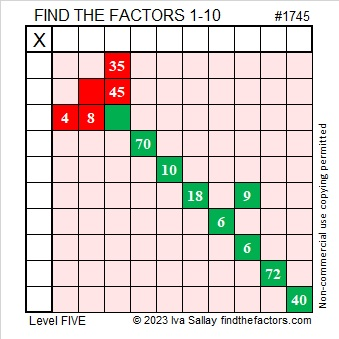Here’s the same puzzle minus the color: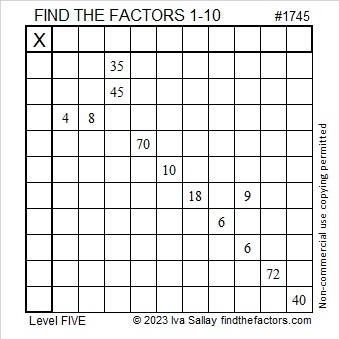### Factors of 1745:

• 1745 is a composite number.
• Prime factorization: 1745 = 5 × 349.
• 1745 has no exponents greater than 1 in its prime factorization, so √1745 cannot be simplified.
• The exponents in the prime factorization are 1 and 1. Adding one to each exponent and multiplying we get (1 + 1)(1 + 1) = 2 × 2 = 4. Therefore 1745 has exactly 4 factors.
• The factors of 1745 are outlined with their factor pair partners in the graphic below.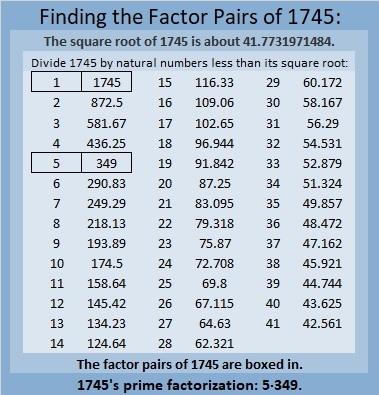### More About the Number 1745:

1745 is the difference of two squares in two different ways:
873² – 872² = 1745, and
177² – 172² = 1745.

1745 is also the sum of two squares in two different ways:
41² + 8² = 1745, and
31² + 28² = 1745.

1745 is the hypotenuse of FOUR Pythagorean triples:
177-1736-1745, calculated from 31² – 28², 2(31)(28), 31² + 28²,
656-1617-1745, calculated from 2(41)(8), 41² – 8², 41² + 8²,
900-1495-1745, which is 5 times (180-299-349)
1047-1396-1745, which is (3-4-5) times 349.

# 1744 XOXO Kisses or Multiplication? XOXO

### Today’s Puzzle:

When I was a little girl, my mother told me that OXOXOX stands for Hugs and Kisses. That would mean that O means “hug” and X means “kiss”, but I think X also means multiplication. It’s almost Valentine’s Day, so in today’s puzzle, it can mean either one.

This is a Level-4 puzzle so the clues don’t come in any particular order. Use logic to place all the numbers 1 to 10 in the first column and in the top row.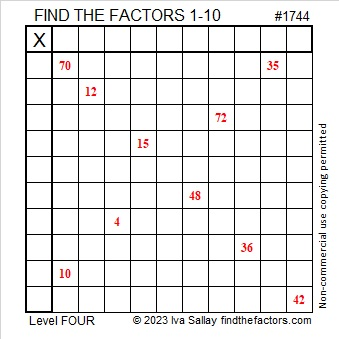### Factors of 1744:

• 1744 is a composite number.
• Prime factorization: 1744 = 2 × 2 × 2 × 2 × 109, which can be written 1744 = 2⁴ × 109.
• 1744 has at least one exponent greater than 1 in its prime factorization so √1744 can be simplified. Taking the factor pair from the factor pair table below with the largest square number factor, we get √1744 = (√16)(√109) = 4√109.
• The exponents in the prime factorization are 4 and 1. Adding one to each exponent and multiplying we get (4 + 1)(1 + 1) = 5 × 2 = 10. Therefore 1744 has exactly 10 factors.
• The factors of 1744 are outlined with their factor pair partners in the graphic below.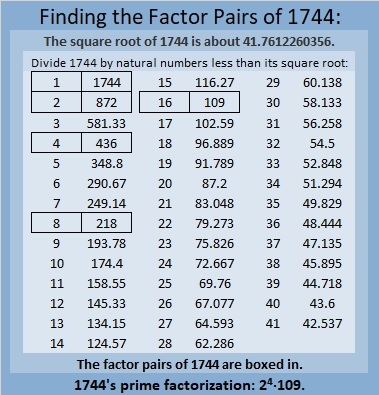### More About the Number 1744:

1744 is the difference of two squares in three ways:
437² – 435² =1744,
220² – 216² =1744, and
113² – 105² =1744.

1744 is also the sum of two squares:
40² + 12² = 1744.

1744 is the hypotenuse of a Pythagorean triple:
960-1456-1744, calculated from 2(40)(12), 40² – 12², 40² + 12².
It is also 16 times (60-91-109).